# The Quadratic Programming Solver

### Example 9.1 Linear Least Squares Problem

The linear least squares problem arises in the context of determining a solution to an overdetermined set of linear equations. In practice, these equations could arise in data fitting and estimation problems. An overdetermined system of linear equations can be defined as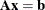where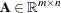,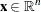,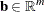, and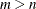. Since this system usually does not have a solution, you need to be satisfied with some sort of approximate solution. The most widely used approximation is the least squares solution, which minimizes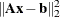.

This problem is called a least squares problem for the following reason. Let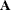,, and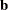be defined as previously. Let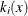be the kth component of the vector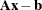: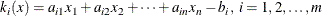By definition of the Euclidean norm, the objective function can be expressed as follows: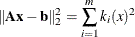Therefore, the function you minimize is the sum of squares of m terms; hence the term least squares. The following example is an illustration of the linear least squares problem; that is, each of the terms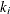is a linear function of x .

Consider the following least squares problem defined by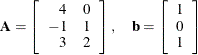This translates to the following set of linear equations: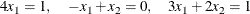The corresponding least squares problem is: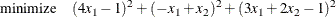The preceding objective function can be expanded to: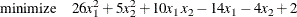In addition, you impose the following constraint so that the equation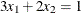is satisfied within a tolerance of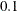: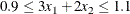You can use the following SAS statements to solve the least squares problem:

/* example 1: linear least-squares problem */
proc optmodel;
var x1; /* declare free (no explicit bounds) variable x1 */
var x2; /* declare free (no explicit bounds) variable x2 */
/* declare slack variable for ranged constraint */
var w >= 0 <= 0.2;

/* objective function: minimize is the sum of squares */
minimize f = 26 * x1 * x1 + 5 * x2 * x2 + 10 * x1 * x2
- 14 * x1 - 4 * x2 + 2;

/* subject to the following constraint */
con L: 3 * x1 + 2 * x2 - w = 0.9;

solve with qp;

/* print the optimal solution */
print x1 x2;
quit;


The output is shown in Output 9.1.1.

Output 9.1.1: Summaries and Optimal Solution

The OPTMODEL Procedure

Problem Summary
Objective Sense Minimization
Objective Function f

Number of Variables 3
Bounded Above 0
Bounded Below 0
Bounded Below and Above 1
Free 2
Fixed 0

Number of Constraints 1
Linear LE (<=) 0
Linear EQ (=) 1
Linear GE (>=) 0
Linear Range 0

Constraint Coefficients 3

Performance Information
Execution Mode Single-Machine
Number of Threads 4

Solution Summary
Solver QP
Algorithm Interior Point
Objective Function f
Solution Status Optimal
Objective Value 0.0095238095

Primal Infeasibility 9.742207E-14
Dual Infeasibility 1.305621E-9
Bound Infeasibility 5.782412E-18
Duality Gap 4.9397126E-8
Complementarity 1.5357015E-7

Iterations 3
Presolve Time 0.00
Solution Time 0.02

x1 x2
0.2381 0.1619